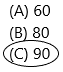# Texas Go Math Grade 2 Lesson 1.2 Answer Key Using Number Lines

Refer to our Texas Go Math Grade 2 Answer Key Pdf to score good marks in the exams. Test yourself by practicing the problems from Texas Go Math Grade 2 Lesson 1.2 Answer Key Using Number Lines.

## Texas Go Math Grade 2 Lesson 1.2 Answer Key Using Number Lines

Explore

Draw points to model the problem.

Jack’s Number Line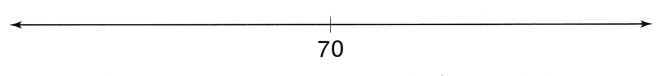Audrey’s Number Line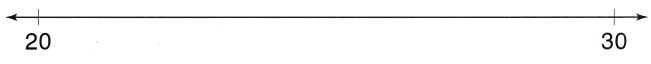FOR THE TEACHER • Read the following to children. Jack wants to draw a point on his number line for a number greater than 70. Audrey wants to draw a point on her number line to show where 22 belongs. Draw to show points that Jack and Audrey could draw.
Number line: A number line is a pictorial representation of numbers on a straight line. It’s a reference for comparing and ordering numbers. It can be used to represent any real number that includes every whole number and natural number. Just to recollect, the whole number is a set of numbers that include all counting numbers (1, 2, 3,4,5,6 …….) and zero (0), whereas the natural number is the set of all counting numbers i.e. 1, 2, 3, 4, 5, 6……..
Jack’s Number line:
Jack’s should draw the number line greater than 70. This number line will represent as: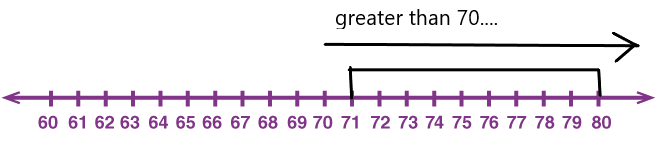Audrey should point out the 22 on the number line: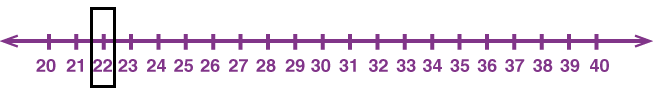Math Talk
Mathematical Processes

Explain how you decided where Jack and Audrey could draw their points.
Answer: Arithmetic operations of numbers can be better explained on a number line. To begin with, one must know to locate numbers on a number line. According to the question we need to point the numbers. number lines are the horizontal straight lines in which the integers are placed in equal intervals. All the numbers in a sequence can be represented in a number line. This line extends indefinitely at both ends.
Here Jack should point the numbers more than 70. So we should place the numbers on the number line. For example, I had placed numbers from 60 to 80 then easily we can point out the numbers that are greater than 70.
Now coming to Audrey, he should point to the number 22. So the number line starts from 20 and the sequence will go on.

Model and Draw

Write the number that belongs at each point.Each line is counting by ones this means we have to add 1 to every next number. By using this technique we can write the numbers on the number line.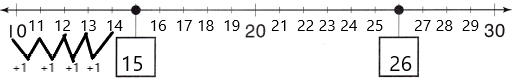Draw and label a point to show where 40 belongs.
Draw and label a point to show where 14 belongs.By using the number line we have to point the numbers. Here is one number line, we have to point to the number 40.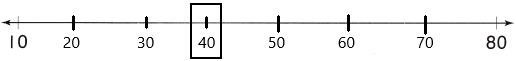Here we have count by 10’s.
Now we have to point to the number 14.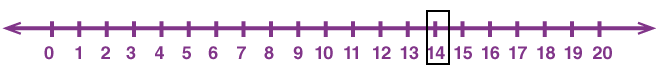Here we have to count by one’s to label the number 14 in the number line.

Share and Show

Question 1.
Write the number that belongs at each point.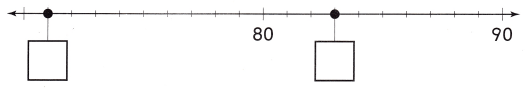count by one to every next number and label the points.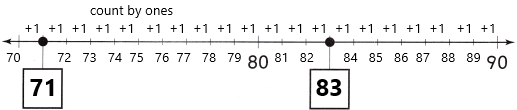Explanation: A number line can be used to represent any real numbers that include whole numbers, whole numbers means a set of numbers that include all zero (0) and counting numbers i.e,1, 2, 3, 4, 5, 6, 7, ….. and so on. Pictorial representation of numbers on a straight line is called Number Line.

Question 2.
Draw and label a point to show where 52 belongs.
Draw and label a point to show where 88 belongs.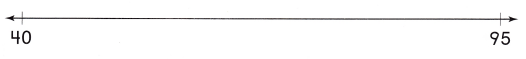A line with numbers placed in their correct position.
First one: We have to draw a number point labelling the number 52. The number line was represented below labelling the number 52.Second one: We have to draw a number point labelling the number 88. The number line was represented below labelling the number 88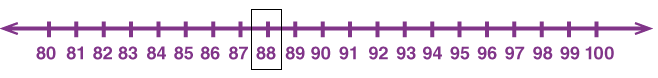To make a number line or to plot a number on it, follow the steps given below:
1. Step 1: Draw a horizontal line with arrows on both ends. [You can also draw a vertical number line]
2. Step 2: Choose a scale depending on the given number. For example, if you have to plot 5, then you can choose a scale of 1. If you have to plot 50, you can choose a scale of either 5, 10, or 25. [Determining an appropriate scale depends on the factors of the given number]
3. Step 3: Mark the points at equal intervals. So, if you have chosen 1 as the scale, then mark 0, 1, 2, 3, 4, and so on.
4. Step 4: Locate the given number. Highlight that point with a circle.

Problem Solving

Question 3.
Write the number that belongs at each point.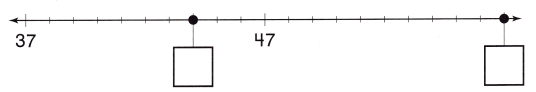Explanation:
1. Step 1: Draw a horizontal line with arrows on both ends. [You can also draw a vertical number line]
2. Step 2: Choose a scale depending on the given number. Already given from 37 from that we need to count and label the number 44 and scale goes on up to 47 which is given already from that we need to count up to 57 and then scale ends in this particular question. [Determining an appropriate scale depends on the factors of the given number]
3. Step 3: Mark the points at equal intervals. So, if you have chosen 1 as the scale, then mark 37, 38, 39, 40, 41, and so on.
4. Step 4: Locate the given number. Highlight that point with a circle.

Question 4.
Draw and label points to show where 10, 17, and 100 belong.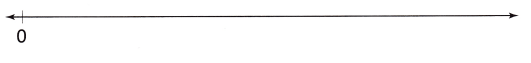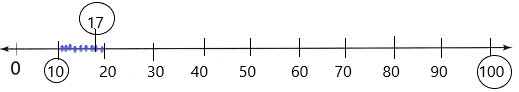Step 1: Draw a horizontal line with arrows on both ends. [You can also draw a vertical number line]
Step 2: Now using the technique count by 10’s we have labelled the numbers up to 100.
Step 3: Now we have to point the given numbers on the number line. Already we have 10 on the number line so round it. To round the 17 counts the blue lines present on the number line and then mark the 17. And the process continues to 100.
Step 4: Locate the given number. Highlight that point with a circle.

Solve.

Question 5.
H.O.T. Multi-Step Choose three of the numbers. Draw and label points for those numbers on the number line.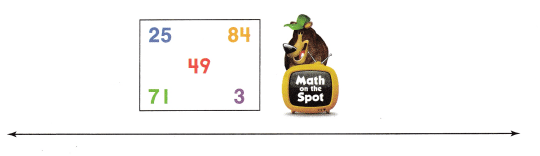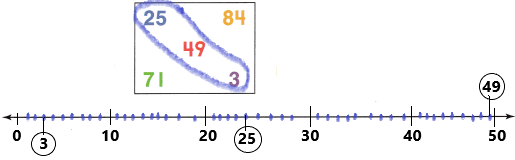Explanation:
Step 1: Draw a horizontal line with arrows on both ends. [You can also draw a vertical number line]
Step 2: Now I choose three of the numbers that are 3, 25, and 49 to the label on the number line.
Step 3: I use a count by 10’s technique and according to that I placed numbers up to 50. And then label the given points on the number line counting blue lines.
Step 4: Locate the given number. Highlight that point with a circle.

Question 6.
H.O.T. Multi-Step Choose one of these points to be the number 30. What might the other points be? Write the numbers.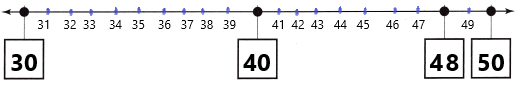Explanation:
Step 1: Draw a horizontal line with arrows on both ends. [You can also draw a vertical number line]
Step 2: Already given the number 30 which we can place in any box. And I choose to keep in the first box and from that, we need to fill another three boxes. So I had placed blue lines from that we can easily fill the boxes.
Step 3: Now count the blue lines according to that write the numbers.
Step 4: Locate the given number. Highlight that point with a circle.

Question 7.
Connect Angel drew this point on the number line to mark his favorite number. Which could be Angel’s favorite number?(A) 12
(B) 15
(C) 6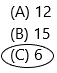Explanation:
Step 1: The given number line is having 0 and 20 numbers and also a dot placed near zero.
Step 2: Now place lines from 1 and keep the lines up to the 19th number.
Step 3: Mark the number which is placed under the dot.Step 4: I got 6 under the dot. So highlight the number.

Question 8.
Analyze Henry’s favourite number is 40. Which point on the number line shows where 40 belongs?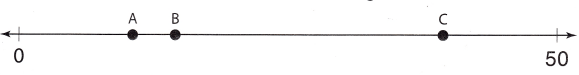(A) point B
(B) point A
(C) point CExplanation:
Step1: The given number line is having 0 and 50 and there are 3 points named with A, B, and C.
Step2: We need to label the number 40 on the number line.
Step 3. Think a while, the number 40 is nearest to 50 so definitely Point C represents the number 40.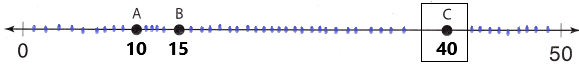Step 4: Locate the number and highlight it with the box.

Question 9.
Does TEXAS Test Prep What number belong at the point?(A) 45
(B) 54
(C) 48Explanation:
Step 1: Observe the number line and the numbers present.
Step 2: There are two numbers 55 and 65. There is a dot which we need to write the correct number under the dot.
Step 3: Count the lines from starting or else we can count the numbers from 55 means in reverse order. 55, 54, 53, 52, 51, 50, 49, 48, 47, 46, 45.Step 4: The dot belongs to the number 48 so mark the number and highlight with the circle.

TAKE HOME ACTIVITY • Have your child explain how he or she solved one of the exercises in this lesson.

### Texas Go Math Grade 2 Lesson 1.2 Homework and Practice Answer Key

Question 1.
Write the number that belongs at each point.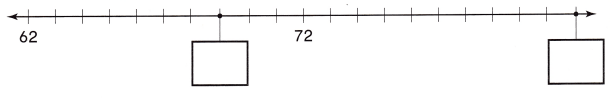Answer: Representation will be shown below: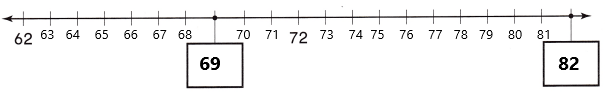Definition of number line: A visual representation of numbers on a straight line drawn either horizontally or vertically is known as a number line. Writing down numbers on a number line makes it easy for us to compare them and to perform basic arithmetic operations on them. There are three parts of a number line which are – negative side, zero, and positive side. Numbers to the left of 0 are negative numbers and numbers to the right of 0 are all positive numbers. So, we can say that on a number line, the numbers present on the right are larger than the numbers on their left.
How to draw a number line:
Step 1: Draw a horizontal line with arrows on both ends. [You can also draw a vertical number line].
Step 2: Choose a scale depending on the given number. In the above problem, the sequence is counting by 1’s.  [Determining an appropriate scale depends on the factors of the given number]
Step 3: Mark the points at equal intervals. So, if you have chosen 1 as the scale, then mark 62, 63, 64, 65 and so on.
Step 4: Locate the given number. Highlight that point with a circle.

Question 2.
Draw and label a point to show where 37 belongs. Draw and label a point to show where 48 belongs.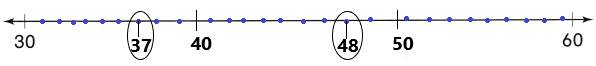Explanation:
Step 1: Draw a horizontal line with arrows on both ends. [You can also draw a vertical number line].
Step 2: Choose a scale depending on the given number. In the above problem, the sequence is counting by 1’s.  [Determining an appropriate scale depends on the factors of the given number]
Step 3: Mark the points at equal intervals. So, if you have chosen 1 as the scale, then mark 37 and 48.
Step 4: Locate the given number. Highlight that point with a circle.

Problem Solving

Solve.

Question 3.
Multi-Step Choose three of the numbers. Draw and label points for those numbers on the number.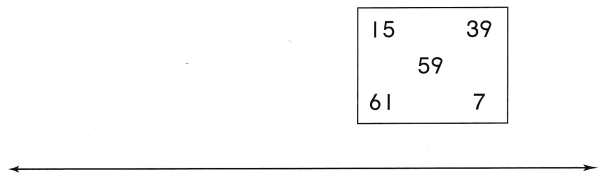Explanation:
Step 1: Draw a horizontal line with arrows on both ends. [You can also draw a vertical number line]
Step 2: Now I choose three of the numbers that are 7, 15, and 39 to the label on the number line.
Step 3: I use a count by 10’s technique and according to that I placed numbers up to 40. And then label the given points on the number line counting blue lines.
Step 4: Locate the given number. Highlight that point with a circle.

Lesson Check

Question 4.
It is 10 steps from the front door of Joke’s house to the sidewalk. Joke walks 7 steps from the door to his bike. Which point could show where Joke’s bike is?(A) Point C
(B) Point B
(C) Point AExplanation:
Step 1: Observe the number line and the points named with A, B, and C.
Step 2: We have to label the number 7 on the number line and which letter represents the number 7.
Step 3: Total 10 steps from door to sidewalk. So according to that, we have to place the numbers.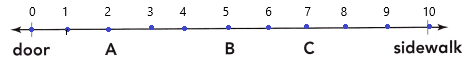Step 4: I marked the number 7 above the letter C.

Question 5.
Maura’s favorite number is 19. Which point on the number line shows where 19 belongs?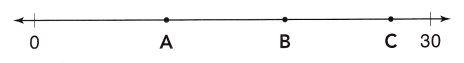(A) Point A
(B) Point B
(C) Point C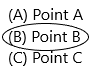Explanation:
Step 1: Draw a horizontal line with arrows on both ends. [You can also draw a vertical number line]
Step 2: we have to find the number 19 and that belongs to a particular letter.
Step 3: I use a count by 1’s technique and according to that I placed numbers up to 30. And then label the given points on the number line counting blue lines. I got 19 on letter B.Step 4: Locate the given number. Highlight that point with a circle.

Question 6.
Look at the points for the numbers 0, 20, and 100. What number might the missing point be?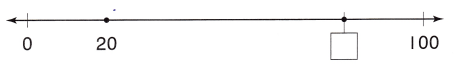(A) 60
(B) 80
(C) 90